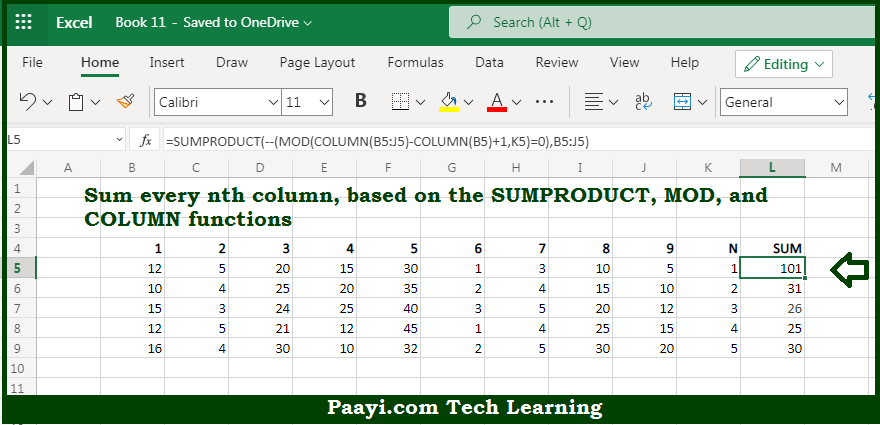# Learn How to SUM Every nth Column in Microsoft Excel

Written by | 0 Comments | 759 Views

In this article, you will learn how to SUM various things in Microsoft Excel using a single/combination(s) of functions. You will also know how to SUM Every nth Column and see the generic formula.

SUM Every nth Column in Microsoft Excel

The main purpose of this formula is to sum every nth column. Here we will learn how to sum every n column in the workbook in Microsoft Excel. That implies, with the help of a formula based on the SUMPRODUCT and MOD function you can able to sum every nth column. So, with the help of this formula, you can able to sum every n column in the workbook in Microsoft Excel.

General Formula to SUM Every nth Column

=SUMPRODUCT(--(MOD(COLUMN(rng)-COLUMN(rng.first)+1,n)=0),rng)

The Explanation for the SUM Every nth ColumnSo we know that with the help of the given formula above you can able sum every nth column. Here we will learn how to sum every n column in the workbook in Microsoft Excel. As we know that the uses SUMPRODUCT function to sum values in a row that have been "filtered" using logic based on MOD function. It should be noted that for the zeros that appear in the columns, the formula uses =0 to force a TRUE when the remainder is zero. Similarly returns FALSE when not, then we use a double-negative (--) to coerce TRUE and FALSE to ones and zeros. So, with the help of this formula, you can able to sum every n column in the workbook in Microsoft Excel.